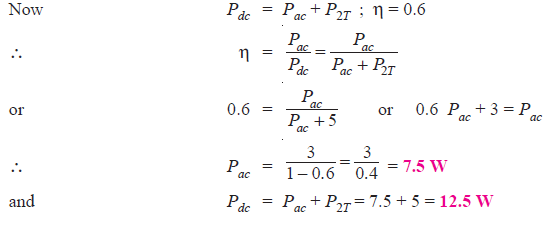Q1. If in Fig. 1; R1 = 10 kΩ ; R2 = 2.2 kΩ ; RC = 3.6 kΩ ; RE = 1.1. kΩ and VCC = + 10 V, find the d.c. power drawn from the supply by the amplifier.

Solution:

The current I1 flowing through R1 also flows through R2 ( assumption because IB is small).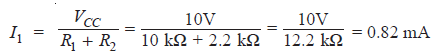D.C. voltage across R2, V2 = I1 R2 = 0.82 mA × 2.2 kΩ = 1.8V
D.C. voltage across RE, VE = V2 – VBE = 1.8V – 0.7V = 1.1V
D.C. emitter current, IE = VE/RE = 1.1V/1.1 kΩ = 1 mA
∴ IC ≅ IE = 1 mA
Total d.c current IT drawn from the supply is;
IT = IC + I1 = 1 mA + 0. 82 mA = 1.82 mA
∴ D.C. power drawn from the supply is
Pdc = VCC IT = 10V × 1.82 mA = 18.2 mW

Q2. Determine the a.c. load power for the circuit shown in Fig. 2.

Solution :

The reading of a.c. voltmeter is 10.6V. Since a.c. voltmeters read r.m.s. voltage, we have,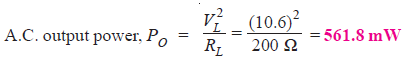Q3. In an RC coupled power amplifier, the a.c. voltage across load RL (= 100 Ω ) has a peak- to-peak value of 18V. Find the maximum possible a.c. load power.

Solution :

The peak-to-peak voltage, VPP = 18V.

Therefore, peak voltage (or maximum voltage) = VPP/2 and the r.m.s value, VL = VPP/2√2 .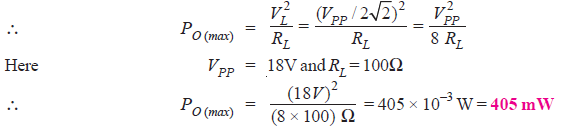Q4. A power amplifier operated from 12V battery gives an output of 2W. Find the maximum collector current in the circuit.

Solution :

Let IC be the maximum collector current.
Power = battery voltage × collector current
or       2 = 12 × IC
∴ IC = 2/12 = 1/6 A = 166.7 mA

Q5. A voltage amplifier operated from a 12 V battery has a collector load of 4 kΩ. Find the maximum collector current in the circuit.

Solution :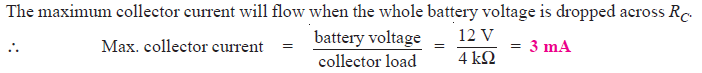Q6. A power amplifier supplies 50 W to an 8-ohm speaker. Find (i) a.c. output voltage (ii) a.c. output current.

Solution :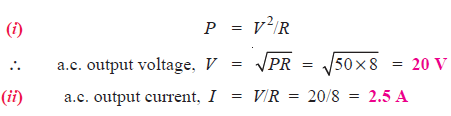Q7. Calculate the (i) output power (ii) input power and (iii) collector efficiency of the amplifier circuit shown in Fig. 3. It is given that input voltage results in a base current of 10 mA peak.

Solution:Fig.3

First draw the d.c. load line by locating the two end points viz.,

IC (sat) = VCC/RC = 20 V/20 Ω = 1 A = 1000 mA

and VCE = VCC = 20 V as shown in Fig. 4.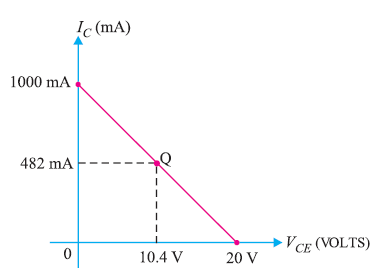The operating point Qof the circuit can be located as under :Q8. A power transistor working in class A operation has zero signal power dissipation of 10 watts. If the a.c. output power is 4 watts, find : (i) collector efficiency (ii) power rating of transistor.

Solution :

Zero signal power dissipation,  Pdc = 10 W

a.c. power output, Po = 4 W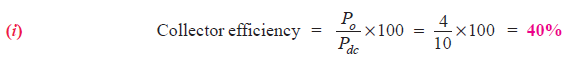(ii) The zero signal power represents the worst case i.e. maximum power dissipation in a transistor occurs under zero signal conditions.

∴ Power rating of transistor = 10 W

It means to avoid damage, the transistor must have a power rating of atleast 10 W.

Q9. A class A power amplifier has a transformer as the load. If the transformer has a turn ratio of 10 and the secondary load is 100 Ω, find the maximum a.c. power output. Given that zero signal collector current is 100 mA.

Solution :

Secondary load, RL = 100 Ω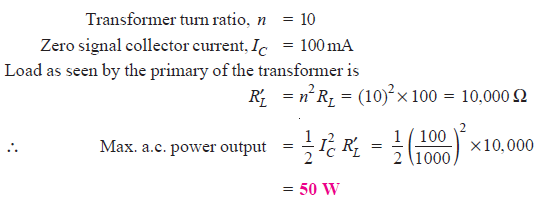Q10.  A class A transformer coupled power amplifier has zero signal collector current of 50 mA. If the collector supply voltage is 5 V, find (i) the maximum a.c. power output (ii) the power rating of transistor (iii) the maximum collector efficiency.

Solution :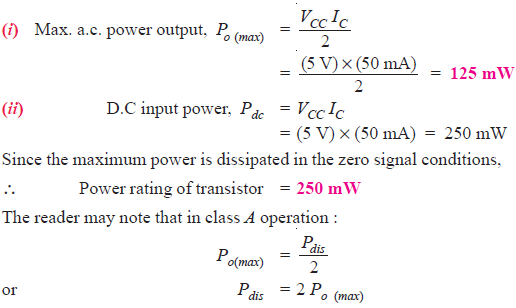It means that power rating of the transistor is twice as great as the maximum a.c. output power. For example, if a transistor dissipates 3 W under no signal conditions, then maximum a.c. output power it can deliver is 1.5 W.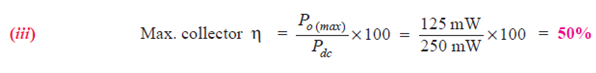Q11. A power transistor working in class A operation is supplied from a 12-volt battery. If the maximum collector current change is 100 mA, find the power transferred to a 5Ω loudspeaker if it is :

(i) directly connected in the collector

(ii) transformer-coupled for maximum power transference

Find the turn ratio of the transformer in the second case.

Solution :(i) Loudspeaker directly connected. Fig.5 shows the circuit of class A power amplifier with loudspeaker directly connected in the collector.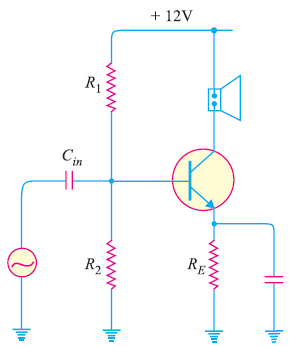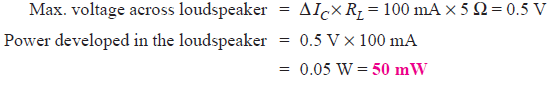Therefore, when loudspeaker is directly connected in the collector, only 50 mW of power is transferred to the loudspeaker.

(ii) Loudspeaker transformer coupled. Fig. 6 shows the class A power amplifier with speaker transformer coupled. As stated before, for impedance matching, step-down transformer is used.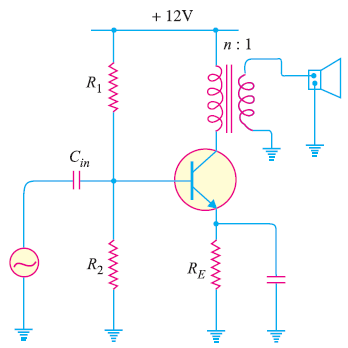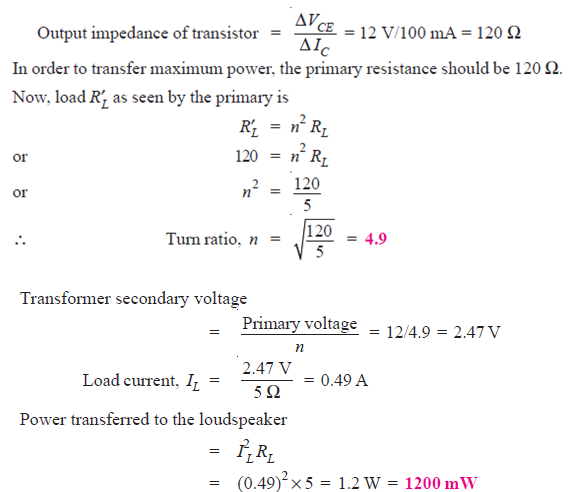Q12. A common emitter class A transistor power amplifier uses a transistor with β =100.The load has a resistance of 81.6 Ω, which is transformer coupled to the collector circuit. If the peak values of collector voltage and current are 30 V and 35 mA respectively and the corresponding minimum values are 5 V and 1 mA respectively, determine :

(i) the approximate value of zero signal collector current

(ii) the zero signal base current

(iii) Pdc and Pac (iv) collector efficiency (v) turn ratio of the transformer.

Solution:

In an ideal case, the minimum values of vCE (min) and iC (min) are zero. However, in actual practice, such ideal conditions cannot be realised. In the given problem, these minimum values are 5 V and 1mA respectively as shown in Fig. 7.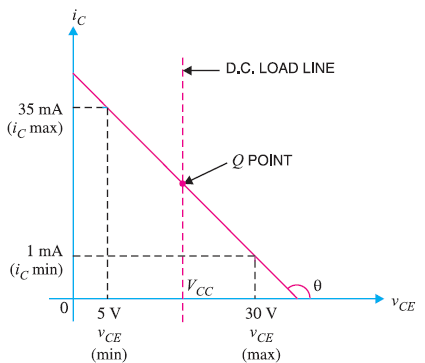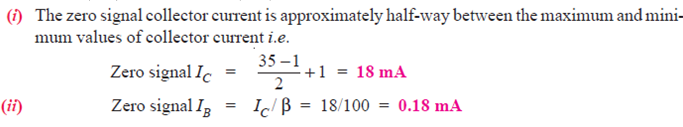Q13. For a class B amplifier using a supply of VCC = 12V and driving a load of 8Ω, determine (i) maximum load power (ii) d.c. input power (iii) collector efficiency.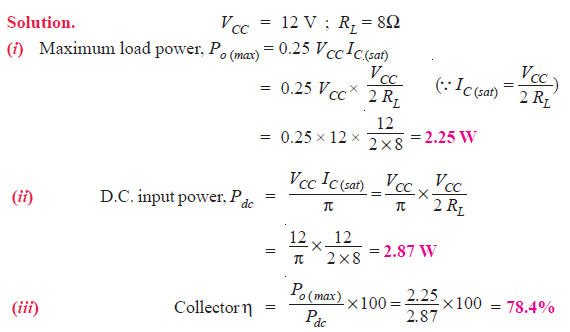Q14. A class B push-pull amplifier with transformer coupled load uses two transistors rated 10 W each. What is the maximum power output one can obtain at the load from the circuit?

Solution :

The power dissipation by each transistor is PT = 10W. Therefore, power dissipated by two transistors is

P2T = 2 × 10 = 20W.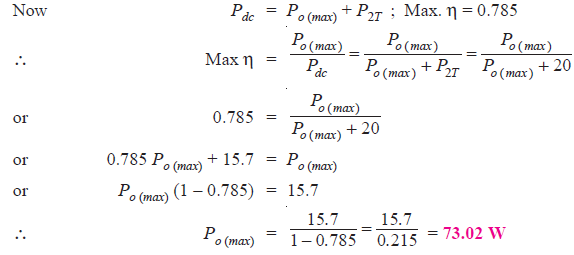Q15. A class B amplifier has an efficiency of 60% and each transistor has a rating of 2.5W. Find the a.c. output power and d.c. input power.

Solution :

The power dissipated by each transistor is PT = 2.5W.

Therefore, power dissipated by the two transistors is P2T = 2×2.5 = 5W.